## which function is equivalent to F(x)=-4(x+7)^2-6?

Question

which function is equivalent to F(x)=-4(x+7)^2-6?

in progress 0
7 months 2021-07-27T14:42:22+00:00 1 Answers 6 views 0

1.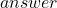Simplify this quadratic function given in vertex form into standard form by simplifying the exponents and adding like terms.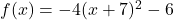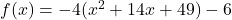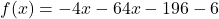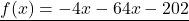(good luck :) and mark me brainliest if youre satisfied with my answer)